## Compute the kinetic energy of a proton (mass 1.67×10−27kg ) using both the nonrelativistic and relativistic expressions for speed of 9.00×10

Question

Compute the kinetic energy of a proton (mass 1.67×10−27kg ) using both the nonrelativistic and relativistic expressions for speed of 9.00×107m/s. Enter your answers numerically separated by a comma.

in progress 0
4 months 2021-09-05T06:28:46+00:00 1 Answers 3 views 0

The non-relativistic kinetic energy of a proton is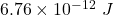The relativistic kinetic energy of a proton is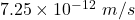Explanation:

Given that,

Mass of proton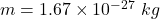Speed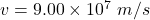We need to calculate the kinetic energy for non relativistic

Using formula of kinetic energy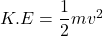Put the value into the formula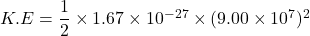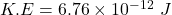We need to calculate the kinetic energy for relativistic

Using formula of kinetic energy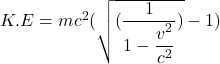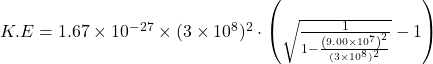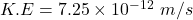Hence, The non-relativistic kinetic energy of a proton isThe relativistic kinetic energy of a proton is